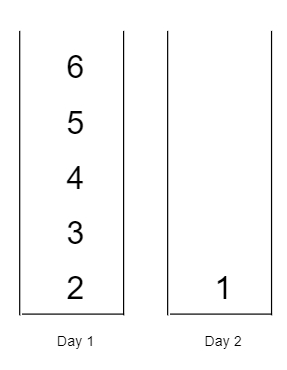# Minimum Difficulty of a Job Schedule in C++

C++Server Side ProgrammingProgramming

Suppose we want to schedule a list of tasks in d days. The tasks are dependent so, to work on the i-th task, we have to finish all the tasks j where 0 <= j < i.

We have to finish at least one task in each day. The difficulty of a task schedule is actually the sum of difficulties of each day of the d number of days. The difficulty of a day is the maximum difficulty of a task that done in that day.

So we have an array of integers called taskDifficulty and an integer d. The difficulty of the i-th job is taskDifficulty[i]. We have to find the minimum difficulty of a task schedule. If we cannot find a schedule for the tasks, then return -1.

So, if the input is like taskDifficulty = [6,5,4,3,2,1], d = 2,then the output will be 7, as on the day 1 we can finish the first 5 jobs, the total difficulty is 6. Now on day 2 we can finish the last job, total difficulty is 1, so the difficulty of the schedule will be 6 + 1 = 7.

To solve this, we will follow these steps −

• Define a function solve(), this will take an array v, idx, k, one 2D dp,

• if idx is same as size of v and k is same as 0, then −

• return 0

• if k < 0 or idx is same as size of v and k > 0, then −

• return 1^6

• if dp[idx, k] is not equal to -1, then −

• return dp[idx, k]

• maxVal := 0

• ret := inf

• for initialize i := idx, when i < size of v, update (increase i by 1), do −

• maxVal := maximum of v[i] and maxVal

• ret := minimum of ret and maxVal + solve(v, i + 1, k - 1, dp)

• dp[idx, k] := ret

• return ret

• From the main method do the following −

• n := size of j

• if d > n, then −

• return -1

• Define one 2D array dp of size n x (d + 1) and fill this with -1

• return solve(j, 0, d, dp)

Let us see the following implementation to get better understanding −

## Example

Live Demo

#include <bits/stdc++.h>
using namespace std;
class Solution {
public:
int solve(vector<int>& v, int idx, int k, vector<vector<int> >&
dp){
if (idx == v.size() && k == 0)
return 0;
if (k < 0 || idx == v.size() && k > 0)
return 1e6;
if (dp[idx][k] != -1)
return dp[idx][k];
int maxVal = 0;
int ret = INT_MAX;
for (int i = idx; i < v.size(); i++) {
maxVal = max(v[i], maxVal);
ret = min(ret, maxVal + solve(v, i + 1, k - 1, dp));
}
return dp[idx][k] = ret;
}
int minDifficulty(vector<int>& j, int d){
int n = j.size();
if (d > n)
return -1;
vector<vector<int> > dp(n, vector<int>(d + 1, -1));
return solve(j, 0, d, dp);
}
};
main(){
Solution ob;
vector<int> v = {6,5,4,3,2,1};
cout << (ob.minDifficulty(v, 2));
}

## Input

{6,5,4,3,2,1}, 2

## Output

7